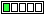All about flooble | fun stuff | Get a free chatterbox | Free JavaScript | Avatarsperplexus dot infoIt is a riddle (Posted on 2005-04-13)It is a riddle.
It is the strongest force,
to resist your force,
and yet it is not a force.
It never accelerates,
but it is always moving.
It never rests, and
it never gets tired.
It has been defined many ways,
but no one can quite capture it.
What is it?

 See The Solution Submitted by Michael Cottle Rating: 3.7143 (14 votes)Comments: ( Back to comment list | You must be logged in to post comments.)re(2): ... (physics 101)| Comment 63 of 87 |(In reply to re: No acceleration from Mathematics point of view by Sam)

To Sam: Amen, mister friend =)

I know everyone will want to hunt me down and shoot me for continuing this pointless topic, but the Earth does accelerate as it moves around the Sun.

The SPEED does not change. That is why the (incorrectly used) equation of a = (V-U)/t ends up equaling 0.

However, the DIRECTION changes. The two components of VELOCITY are speed AND direction. So if one or both of them change, then the velocity has changed and acceleration has occurred. When we have a changing direction, it is helpful to use vector components. A = [V(moving north) � U(moving south-east)]/t doesn�t have any meaning until you break it up into components.

Pretend you are floating out in the universe and can see the "top view" of the earth rotating around the sun. It looks kind of like a clock to me, with the Sun in the center of the clock, and the Earth moving where the ends of the hands of the clock would be. I�ll put my origin of reference at the Sun, and the x-axis points at the 3 and the y-axis points at the 12.

So when the earth is at the 9, it is moving with a speed S and direction 90 degrees. So the x component of the velocity is 0 and the y component is S.

When the earth is at the 12, it is still moving with a speed S, but now the direction is 0 degrees. So now the x component of the velocity is S and the y component is 0.

Now we can compute the average acceleration for this period of time. Ax and Ay mean the x and y components of acceleration. One we find them, A = sqrt(Ax^2 + Ay^2). Ux and Uy just mean the x and y components of earth�s velocity when it is at the 9, and Vx and Vy are the x and y components of the earth�s velocity when it is at the 12. So Ux=0 and Uy=S, and Vx=S and Vy=0.

So we have

Ax = (Vx � Ux)/t = (S-0)/t = S/t
Ay = (Vy � Uy)/t = (0-S)/t = -S/t

A = sqrt(Ax^2 + Ay^2) = sqrt[(S/t)^2 + (-S/t)^2] = sqrt[2(S/t)^2] = sqrt(2)*S/t. Notice this does not equal 0.

Therefore the acceleration is not 0.

And does anyone else actually cringe inside when someone says that if the velocity is 0 then the acceleration is 0? Apparently these people have never tossed a ball in the air. I apologize, I know that sounded very arrogant. But I really think people should study Physics (and pass it) before they use it in their arguments.

 Posted by nikki on 2005-04-29 14:39:07Please log in:
 Login: Password: Remember me: Sign up! | Forgot password

 Search: Search body:
Forums (0)
Newest Problems
Random Problem
FAQ | About This Site
Site Statistics
New Comments (3)
Unsolved Problems
Top Rated Problems
This month's top
Most Commented On

Chatterbox:
Copyright © 2002 - 2021 by Animus Pactum Consulting. All rights reserved. Privacy Information